##### Circuit Analysis For DummiesThe unit step (Heavyside) function models the behavior of a switch (off/on). The unit step function can describe sudden changes in current or voltage in a circuit. The unit step function looks like, well, a step. Practical step functions occur daily, like each time you turn mobile devices, stereos, and lights on and off. Here’s the general definition of the unit step function: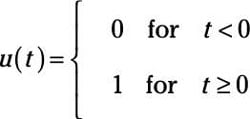So this step function is equal to 0 when time t is negative and is equal to 1 when time t is 0 or positive. Alternatively, you can say there’s a jump in the function value at time t = 0. Math gurus call this jump a discontinuity.

Although you can’t generate an ideal step function, you can approximate a step function. Here is what a step function looks like, along with a circuit that’s roughly a step function.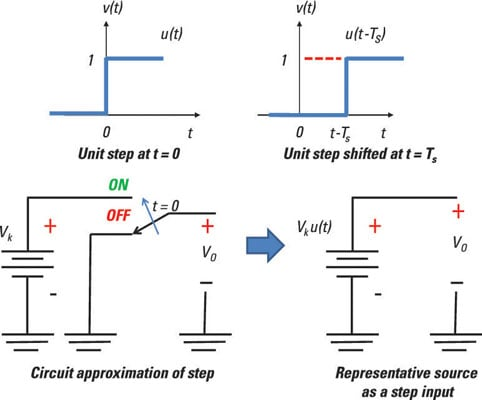## Create a time-shifted, weighted step function

The circuit approximation of the step function shown earlier assumes you can quickly change from off to on at time t = 0 when the switch is thrown.

Although the unit step function appears not to do much, it’s a versatile signal that can build other waveforms. In a graph, you can make the step shrink or stretch. You can multiply the step function u(t) by a constant amplitude Vk to produce the following waveform: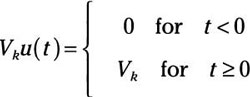The scale or weight of the unit input is Vk. The amplitude Vk measures the size of the jump in function value.

You can move the step function in time with a shift of Ts, leading you to a shifted, weighted waveform: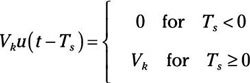This equation says the function equals 0 before time Ts and that the value of the function jumps to Vk after time Ts. Here you see the step function weighted by Vk with a time shift of Ts.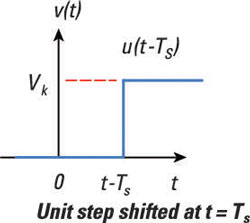You can add two step functions together to form a pulse function, as you learn in the next section.

## Circuit analysis and shifted step functions

Step functions can dance around, but it’s not the fancy twist-and-shout kind of dancing. The function can become bigger or smaller and move to the left or right. You can add those modified step functions to make even more funky step functions.

For example, you can generate a rectangular pulse as a sum of two step functions. Here is a visual of this concept, which shows a rectangular pulse that consists of the sum of two step functions in time.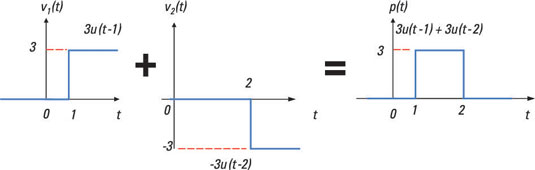Before 1 second, the value of the pulse is 0. Then the amplitude of the pulse jumps to a value of 3 and stays at that value between 1 and 2 seconds. The pulse then returns to 0 at time t = 2 seconds. You wind up with the rectangular pulse p(t) described as the sum of two step functions:

p(t) = 3u(t – 1) – 3u(t – 2)

This expression says that you create a pulse with a time-shifted step function starting at 1 second with an amplitude of 3 and add it to another time-shifted step function starting at 2 seconds with an amplitude of –3. You can view the pulse as a gating function for electronic switches to allow or stop a signal from passing through.

## Build a ramp function with a step function

The integral of the step function generates a ramp function, which consists of two functions multiplied together: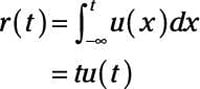The time function tu(t) is simply a ramp function with a slope (or strength) of 1, and the unit step function serves as a convenient mathematical tool to start the ramp at time t = 0. You can add a strength K to the ramp and shift the ramp function in time by TS as follows:

v(t) = Kr(t – TS)

The ramp doesn’t start until TS. Before the time shift TS, the ramp function is 0. After time TS, the ramp has a value equal to Kr(tTS).

With ramp functions, you can create triangular and sawtooth functions (or waveforms). Here you see a ramp of unit strength, a ramp of strength K with a time shift of 1, a triangular waveform, and a sawtooth waveform.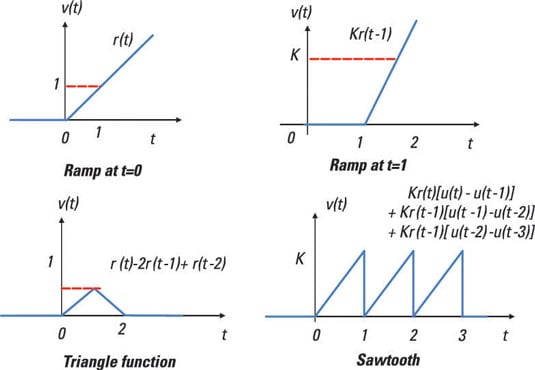Building such waveforms from other functions is useful when you’re breaking the input into recognizable pieces and applying superposition.

Here’s how to build the triangle function shown in the figure, using ramp functions:

1. Turn on a ramp with a slope of 1 starting at time t = 0.

2. Add a ramp that has a slope of –2 and starts at t = 1.

At t = 1, you see the function start to decrease with a slope of –1. But before that, the slope of the function (from the first ramp) is 1; adding a ramp with a slope of –2 to the first ramp results in a ramp with a slope of –1.

3. Turn off the second ramp by adding another delayed ramp that has a slope of 1 and starts at time t = 2.

Adding a ramp with a slope of 1 brings the slope back to 0.

Here’s the math behind this:

v(t) = r(t) – 2r(t – 1) + r(t – 2)

Here’s how to build a sawtooth function like the one shown in the figure, using ramp and step functions:

1. Start with a ramp of slope (or strength) K multiplied by a rectangular pulse of unit height.

The pulse consists of two step functions. Mathematically, you have a ramp with a specific time duration:

r1(t) = Kr(t)[u(t) – u(t – 1)]
2. Apply a time delay of 1 to the ramp pulse r1(t) to get another ramp pulse r2(t) that’s time shifted.

You get the following:

r2(t) = Kr1(t – 1) = Kr(t – 1)[u(t – 1) – u(t – 2)]
3. Repeat Step 2 to get more delayed ramp pulses starting at 2, 3, 4, and so on.

4. Add up all the functions to get the sawtooth st(t).

Here’s the sawtooth function:

st(t) = K{r(t)[u(t) – u(t – 1)] + r(t – 1)[u(t – 1) – u(t – 2)]+…+}